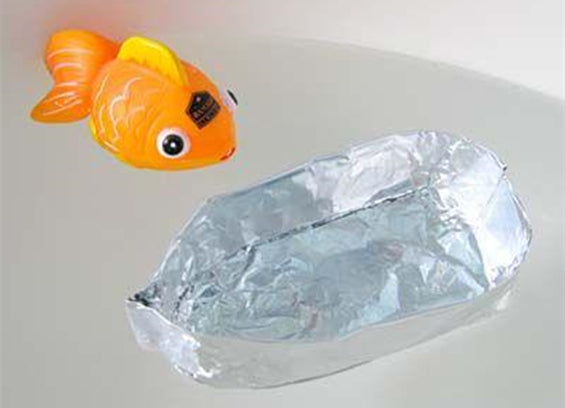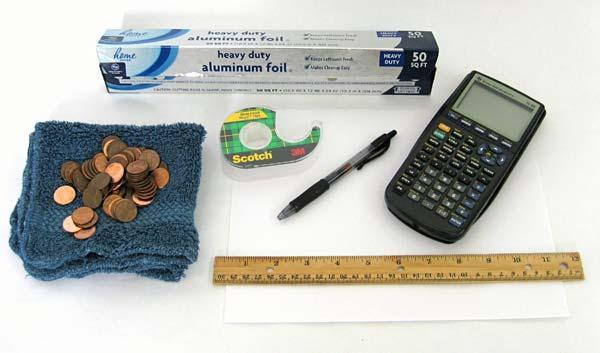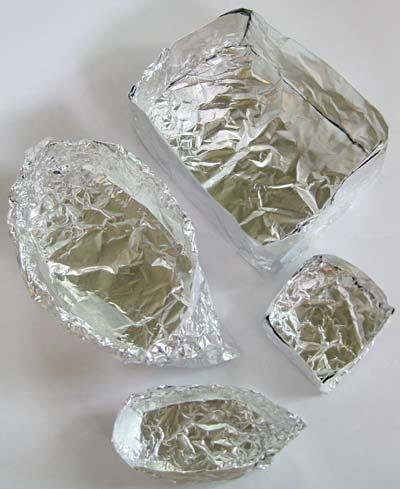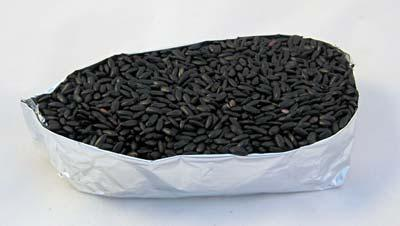## Inspiration# How Much Weight Can Aluminum Foil Boats Float

## Introduction

Have you ever wondered how a ship made of steel can float? If you drop a steel bolt in a bucket of water, the bolt quickly sinks to the bottom. Then how can a steel ship float? And better yet, how can a steel ship carry a heavy load without sinking? It has to do with the density, or the mass per volume, of the ship (and its cargo) compared to the density of water. In this science activity, you will make little "boats" out of aluminum foil to explore how their size affects how much weight they carry and how this relates to the density of water.

## Credits

Teisha Rowland, PhD, Science Buddies

• Aluminum foil
• Ruler
• Tape
• Scrap piece of paper and pen or pencil
• Rag or paper towels
• Pennies. You may need as many as 200 pennies, depending on the size and shape of the boats you make.
• Calculator
• Optional: Dry rice and measuring cup
• Bucket, tub, sink, or dishpan
• Water## Procedure

1. Cut two squares of aluminum foil, making one square have dimensions that are twice that of the other square. For example, you could make one square be 12 inches by 12 inches (or 30 centimeters [cm] by 30 cm), and make the second square be 6 inches by 6 inches (or 15 cm by 15 cm).
2. Fold the two aluminum foil squares into two different boat hulls. Try to make them the same shape. For example, you could make them both have two pointed ends (like canoes) or you could make them square or rectangular (i.e., rectangular prisms).3. Make finishing touches to the boat hulls. Make sure they do not have any leaks. If needed, use a little tape to make them stronger. Flatten the bottoms of the hulls. On each, try to make sure the rim is the same height going all around the edge of the hull.
4. Calculate the volume of each boat hull. If both hulls are rectangular prisms, you can measure the length, width, and height of each hull and multiply these dimensions together to get its volume. If parts of the hull have an irregular shape, measure the volume piece-wise and then add these volumes together. Use triangles to approximate any areas of the hull that are curved or angled.What is the volume of each hull?

5.6. Alternatively, you can use dry rice to calculate the volume of each boat hull. To do this, carefully fill each hull with dry rice so that the rice is level with the top of the hull. Being careful not to damage the hull, transfer the dry rice into a measuring cup. What is the volume of each hull using the rice?7. Fill the bucket, tub, sink, or dishpan with some water.
8. Take one of the boat hulls and carefully float it in the container of water.
9. Gently add one penny at a time. To prevent the hull from tipping, carefully balance the load as you add pennies (left to right, front to back — or port to starboard, fore to aft, if you are feeling nautical).10. Keep adding pennies until the hull finally sinks.
11. Carefully take out the sunken hull and place it and the pennies on a rag or paper towels. Dump any excess water back into the container.
12. Count how many pennies the hull could support before sinking (i.e., the penny that sank the hull does not count).How many pennies could it support?

13. Repeat this process with the other hull. Besure to only add dry pennies. Why do you think using dry pennies (instead of wet ones) is important?
14. Could the larger hull support a lot more pennies than the smaller one?
15. Make sure that the volume you calculated for each boat is in cubic centimeters (cm³). Convert it if necessary.  Tip: Cubic centimeters are the same as milliliters (mL).
16. Convert the number of pennies each hull could support to grams. To do this, multiply the number of pennies by 2.5 grams (the weight of a single penny). How many grams could each hull support?
17. For each hull, divide the number of grams it could support by its volume in cubic centimeters. This roughly gives you the hull's density.What was the density of each hull right before sinking? How do you think this relates to the density of water?

## What Happened?

When you first put one of the boat hulls on the water, it should have floated because its total density (or mass per unit of volume) was less than the density of water. As you added pennies to the hull, its density increased and the hull floated lower. Eventually, when enough pennies were added, the hull's density roughly equaled the density of water. This happens right before the penny is added that sinks the hull. The hull sinks because its density has finally become greater than the density of water. Consequently, the density of the hull right before sinking should roughly equal the density of water, which is 1 gram per cubic centimeter. Even though the larger hull supports more weight, the larger hull also has a larger volume, and both hulls should roughly have a density of 1 gram per cubic centimeter right before sinking. (Your densities may not have been exactly this, but may have ranged between 0.7 to 1.3 grams per cubic centimeter. Sources of error that you could try to get rid of to give you an answer closer to the actual density of water include more accurately calculating the volume of each hull, using something smaller than pennies, and including the hull's weight in your calculations.)

## Digging Deeper

What determines whether an object floats or sinks? It is the density (mass per unit volume) of the object compared to the density of the liquid it is in. If the object is denser than the fluid, the object will sink. If the object is less dense than the fluid, the object will float.

With a steel-hulled ship, it is the shape of the ship's hull that matters. On an empty ship with a steel hull enclosing a volume of air, the ship's density is equal to the sum of the mass of the steel hull and the mass of the enclosed air, all divided by the hull's volume. The ship floats because its density is less than the density of water. But when cargo or other weight is added to the ship, its density now becomes the sum of the mass of the steel hull, enclosed air, and the cargo, all divided by the hull's volume. If too much weight is added, the density of the ship becomes greater than the density of water, and the ship sinks. Extra cargo would need to be thrown overboard in a hurry or it is time to abandon ship!